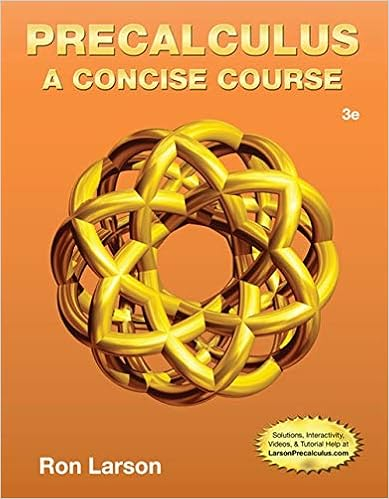# 351table disposable income and spending look at the

• 82
• 94% (34) 32 out of 34 people found this document helpful

This preview shows page 65 - 68 out of 82 pages.

##### We have textbook solutions for you!
The document you are viewing contains questions related to this textbook.The document you are viewing contains questions related to this textbook.
Chapter 2 / Exercise 94
Precalculus: A Concise Course
LarsonExpert Verified
351.(Table: Disposable Income and Spending) Look at the table Disposable Income and Spending. Develop a linear equation of the consumption function. Use this consumption function to forecast the amount of consumption spending that would occur if disposable income were equal to \$500.Page 65
##### We have textbook solutions for you!
The document you are viewing contains questions related to this textbook.The document you are viewing contains questions related to this textbook.
Chapter 2 / Exercise 94
Precalculus: A Concise Course
LarsonExpert Verified
Use the following to answer question 352:352.(Table: Real GDP) Suppose the economy has no government spending and no foreign trade. With no taxes and transfers, real GDP equals disposable income (YD). The data in the accompanying table show consumption spending (C) and planned investment (IPlanned).a. What is the marginal propensity to consume in this economy?b. At what level of real GDP will the economy find its income–expenditure equilibrium?353.The multiplier process:A)explains how spending continues indefinitely with continuous rounds of spending.B)ends after one round of spending and with total spending limited to the initial changein spending.C)occurs only when economies are in an expansion phase.D)is limited, with the total change in real GDP dependent upon the size of the marginalpropensity to consume.354.The value of the multiplier will be smaller:A)the larger the value of the marginal propensity to save.B)the larger the value of the marginal propensity to consume.C)if the marginal propensity to consume equals the marginal propensity to save.D)if the marginal propensity to consume plus the marginal propensity to save equals 1.355.During the Great Depression:A)investment fell, but consumption increased.B)investment increased, but consumption decreased.C)both consumption and investment decreased.D)overall GDP rose.356.All of the following affect consumer spending EXCEPT:Page 66
A)current disposable income.B)wealth.C)past disposable income.D)expected disposable income.357.Two-thirds of total spending is usually attributed to:A)consumption.B)investment.C)government spending.D)net exports.358.The marginal propensity to save:A)is the change in consumer savings divided by the change in consumption.B)is the change in savings divided by the change in disposable income.C)equals MPC+ 1.D)changes when the marginal propensity to consume is constant.359.Alice's disposable income increases by \$1,000, and she spends \$600 of it. Assuming no taxes, Alice's:A)marginal propensity to save is 0.4 and she saves \$400.B)MPCis 0.4 and she saves \$400.C)marginal propensity to save is 0.4 and she saves \$600.D)MPCis 0.6 and she consumes \$400.360.When Julie Ann's disposable income is \$10,000, she spends \$10,000, and when her disposable income is \$15,000, her spending is \$12,500. Julie Ann's autonomous consumption is _____ and her marginal propensity to consume is 0.5.A)\$5,000B)\$10,000C)\$15,000D)\$0361.The slope of the consumption function equals:A)1 – MPS.B)1 / (1 – MPS).C)1 – MPC.D)MPC / MPS.362.If housing prices rise nationwide, there will be a(n):A)increase in consumer spending at any given level of disposable income.
•••